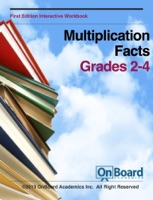# Multiplication Facts Book Details

## By Todd Deluca

• Category: Mathematics, Books, Science & Nature
• Type: ebook
• Release Date: 2013-04-10
• Author: Todd Deluca
• Book Ratings: 4.5/5 (5 User Reviews)
Score Ratings: 4.5
4.5
Based on 5 User Reviews

Get a Copy :

...

### Multiplication Facts Overview

Interactive Workbook includes interactive activities and a companion quiz.  This work book is intended to be instructor lead.  Instructors guide is included.

Lesson Objective

Multiplication facts (e.g. 4 groups of 3 is equal to 3 groups of 4)

Common Core Alignment

Operations & Algebraic Thinking

Represent and solve problems involving multiplication and division.

3.OA.1. Interpret products of whole numbers, e.g., interpret 5 × 7 as the total number of objects in 5 groups of 7 objects each. For example, describe a context in which a total number of objects can be expressed as 5 × 7.

3.OA.3.  Use multiplication and division within 100 to solve word problems in situations involving equal groups, arrays, and measurement quantities, e.g., by using drawings and equations with a symbol for the unknown number to represent the problem.

3.OA.4.  Determine the unknown whole number in a multiplication or division equation relating three whole numbers. For example, determine the unknown number that makes the equation true in each of the equations 8 × ? = 48, 5 = _ ÷ 3, 6 × 6 = ?

Operations & Algebraic Thinking

Represent and solve problems involving multiplication and division.

Understand properties of multiplication and the relationship between multiplication and division.

3.OA.5.  Apply properties of operations as strategies to multiply and divide. Examples: If 6 × 4 = 24 is known, then 4 × 6 = 24 is also known. (Commutative property of multiplication.) 3 × 5 × 2 can be found by 3 × 5 = 15, then 15 × 2 = 30, or by 5 × 2 = 10, then 3 × 10 = 30. (Associative property of multiplication.) Knowing that 8 × 5 = 40 and 8 × 2 = 16, one can find 8 × 7 as 8 × (5 + 2) = (8 × 5) + (8 × 2) = 40 + 16 = 56. (Distributive property.)

Multiply and divide within 100.

3.OA.7. Fluently multiply and divide within 100, using strategies such as the relationship between multiplication and division (e.g., knowing that 8 × 5 = 40, one knows 40 ÷ 5 = 8) or properties of operations. By the end of Grade 3, know from memory all products of two one-digit numbers.

Solve problems involving the four operations, and identify and explain patterns in arithmetic.

3.OA.9.  Identify arithmetic patterns (including patterns in the addition table or multiplication table), and explain them using properties of operations. For example, observe that 4 times a number is always even, and explain why 4 times a number can be decomposed into two equal addends.

Operations & Algebraic Thinking

Use the four operations with whole numbers to solve problems.

4.OA.1. Interpret a multiplication equation as a comparison, e.g., interpret 35 = 5 × 7 as a statement that 35 is 5 times as many as 7 and 7 times as many as 5. Represent verbal statements of multiplicative comparisons as multiplication equations.

1431 Comments